## In a wire AB, 25*10^21 electrons move from point A and B in 100 seconds. What is the current between A and B? What is the direction of this

Question

In a wire AB, 25*10^21 electrons move from point A and B in 100 seconds. What is the current between A and B? What is the direction of this current?

in progress 0
2 months 2021-08-01T04:18:48+00:00 1 Answers 1 views 0

40 A, current is from B to A

Explanation:

The current in a wire is defined as: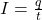where

q is the amount of charge passing a given point of the wire in a time interval t

The charge passing through a given point can be written as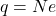where

N is the number of electrons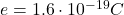is the charge of one electron

So we can rewrite the equation as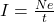In this problem: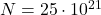is the number of electrons

t = 100 s is the time interval

Substituting, we find the current: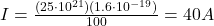Moreover, the direction of a current is defined as the direction of positive charges moving. Here, the charge carriers are electrons (which are negatively charged), so the direction of the current is opposite to the direction of motion of the electrons: therefore, since the electrons move from A to B, the direction of the current is from B to A.